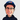### Dang it# Algorithms: Big-O Notation

May 15, 2019 - 3 min read 🍵🍵

Tags: computer scienceActive recall is an effective learning technique to help solidify the material learned by actively engaging your memory. It is a proven technique to help you learn new material. As someone with a science background that has no relevance in Computer Science, I will be using this technique to learn concepts that I need to become a developer.

Below are my active recall questions. Materials have been taken from either the textbook, Introduction to Algorithms 3rd Edition by Thomas H. Cormen et al. or from the MIT course.

## What is an Algorithm?

An algorithm is the sequence of computational steps that transforms input into output.

## Why do we care about Algorithms?

We care about algorithms because computing is time bounded and so are the resources (e.g space in memory). Algorithms can solve all sorts of problems such as identifying the genes in a human genome and determining sequences of base pairs, finding the shortest distance on a map, automation and any problem that can be solved with step by step instructions to produce a result.

It is important to be able to develop different algorithms to tackle different problems. Overtime, developing a pattern can help us solve a similar problem we will encounter in the future.

I’ve used a Python course I found here with interactive examples to help me learn this concept as well as this YouTube video to explain. I found that the textbook gets really in depth with the technical side, using other resources helped clear any confusion I had.

## Explain the Big-O Notation

An algorithm can be broken down into different time complexities which can be explained in a systematic and mathematical way, hence, the Big-O Notation. There are common functions for the Big-O which will be explored throughout this journey.

It is written as a function T(n) = 1 + n, where T is the time it takes to run a function, grows as the size of the input n grows. From what I understand, the problem of an algorithm become larger due to the number of inputs. There will be some part of the formula that can provide a useful approximation to the actual number of steps in computation.

Therefore, when comparing algorithms, we can directly compare them using the Big-O notation. This will explain how long it takes to complete its algorithm with respect to input size when it becomes infinitely large.

My goal here is to understand the fundamentals of Algorithms and Data Structures because the entire premise of programming is the process of taking an algorithm to provide a solution. Therefore, I feel that it is important to know this topic regardless of how I’m able to program any projects. I can say that I’ve learned this topic well enough to understand the term and theory behind Big-O notation.A blog by Kien